# Analytical Reasoning Quiz I, Non Verbal Reasoning

## 15 Questions MCQ Test Non Verbal Reasoning | Analytical Reasoning Quiz I, Non Verbal Reasoning

Description
Attempt Analytical Reasoning Quiz I, Non Verbal Reasoning | 15 questions in 30 minutes | Mock test for LR preparation | Free important questions MCQ to study Non Verbal Reasoning for LR Exam | Download free PDF with solutions
QUESTION: 1

### Find the number of triangles in the given figure.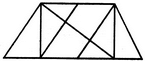Solution:

The figure may be labelled as shown.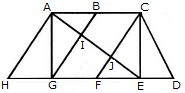The simplest triangles are AHG, AIG, AIB, JFE, CJE and CED i.e. 6 in number.

The triangles composed of two components each are ABG, CFE, ACJ and EGI i.e. 4 in number.

The triangles composed of three components each are ACE, AGE and CFD i.e. 3 in number.

There is only one triangle i.e. AHE composed of four components.

Therefore, There are 6 + 4 + 3 + 1 = 14 triangles in the given figure.

QUESTION: 2

### Find the minimum number of straight lines required to make the given figure.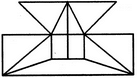Solution:

The figure may be labelled as shown.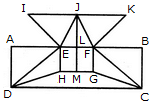The horizontal lines are IK, AB, HG and DC i.e. 4 in number.

The vertical lines are AD, EH, JM, FG and BC i.e. 5 in number.

The slanting lines are IE, JE, JF, KF, DE, DH, FC and GC i.e. 8 is number.

Thus, there are 4 + 5 + 8 = 17 straight lines in the figure.

QUESTION: 3

### Find the number of triangles in the given figure.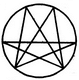Solution:

The figure may be labelled as shown.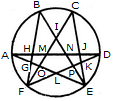The simplest triangles are AGH, GFO, LFO, DJK, EKP, PEL and IMN i.e. 7 in number.

The triangles having two components each are GFL, KEL, AMO, NDP, BHN, CMJ, NEJ and HFM i.e. 8 in number.

The triangles having three components each are IOE, IFP, BIF and CEI i.e. 4 in number.

The triangles having four components each are ANE and DMF i.e. 2 in number.

The triangles having five components each are FCK, BGE and ADL i.e. 3 in number.

The triangles having six components each are BPF, COE, DHF and AJE i.e. 4 in number.

Total number of triangles in the figure = 7 + 8 + 4 + 2 + 3 + 4 = 28.

QUESTION: 4

Find the number of triangles in the given figure.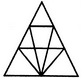Solution:

The figure may be labelled as shown.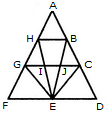The simplest triangles are AHB, GHI, BJC, GFE, GIE, IJE, CEJ and CDE i.e. 8 in number.

The triangles composed of two components each are HEG, BEC, HBE, JGE and ICE i.e. 5 in number.

The triangles composed of three components each are FHE, GCE and BED i.e. 3 in number.

There is only one triangle i.e. AGC composed of four components.

There is only one triangle i.e. AFD composed of nine components.

Thus, there are 8 + 5 + 3 + 1 + 1 = 18 triangles in the given figure.

QUESTION: 5

Find the minimum number of straight lines required to make the given figure.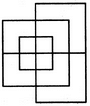Solution:

The figure may be labelled as shown.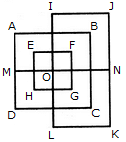The horizontal lines are IJ, AB, EF, MN, HG, DC and LK i.e. 7 in number.

The vertical lines are AD, EH, IL, FG, BC and JK i.e. 6 in number.

Thus, there are 7 + 6 = 13 straight lines in the figure.

QUESTION: 6

Find the number of triangles in the given figure.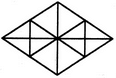Solution:

The figure may be labelled as shown.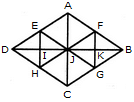The simplest triangles are AFJ, FJK, FKB, BKG, JKG, JGC, HJC, HIJ, DIH, DEI, EIJ and AEJ i.e. 12 in number.

The triangles composed of two components each are JFB, FBG, BJG, JFG, DEJ, EJH, DJH and DEH i.e. 8 in number.

The triangles composed of three components each are AJB, JBC, DJC and ADJ i.e. 4 in number.

The triangles composed of six components each are DAB, ABC, BCD and ADC i.e. 4 in number.

Thus, there are 12 + 8 + 4 + 4 = 28 triangles in the figure.

QUESTION: 7

Find the number of triangles in the given figure.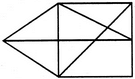Solution:

The figure may be labelled as shown.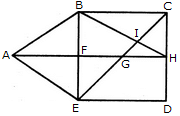The simplest triangles are ABF, BIC, CIH, GIH, FGE and AFE i.e. 6 in number.

The triangles composed of two components each are ABE, AGE, BHF, BCH, CGH and BIE i.e. 6 in number.

The triangles composed of three components each are ABH, BCE and CDE i.e. 3 in number.

Hence, the total number of triangles in the figure = 6 + 6 + 3 = 15.

QUESTION: 8

Find the number of triangles in the given figure.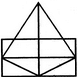Solution:

The figure may be labelled as shown.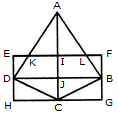The simplest triangles are AKI, AIL, EKD, LFB, DJC, BJC, DHC and BCG i.e. 8 in number.

The triangles composed of two components each are AKL, ADJ, AJB and DBC i.e. 4 in number.

The triangles composed of the three components each are ADC and ABC i.e. 2 in number.

There is only one triangle i.e. ADB composed of four components.

Thus, there are 8+ 4 + 2 + 1= 15 triangles in the figure.

QUESTION: 9

Find the number of triangles in the given figure.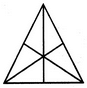Solution:

The figure may be labelled as shown.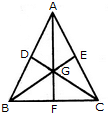The simplest triangles are AGE, EGC, GFC, BGF, DGB and ADG i.e. 6 in number.

The triangles composed of two components each are AGC, BGC and ABG i.e. 3 in number.

The triangles composed of three components each are AFC, BEC, BDC, ABF, ABE and DAC i.e. 6 in number.

There is only one triangle i.e. ABC composed of six components.

Thus, there are 6 + 3 + 6 + 1 = 16 triangles in the given figure.

QUESTION: 10

Find the number of triangles in the given figure.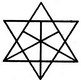Solution:

The figure may be labelled as shown.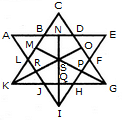The simplest triangles are ABL, BCD, DEF, FGP, PGH, QHI, JQI, KRJ and LRK i.e. 9 in number.

The triangles composed of two components each are OSG, SGQ, SPI, SRI, KSQ, KMS, FGH, JHI and JKL i.e. 9 in number.

There is only one triangle i.e. KSG which is composed of four components.

The triangles composed of five components each are NEI, ANI, MCG and KCO i.e. 4 in number.

The triangles composed of six components each are GMK and KOG i.e. 2 in number.

There is only one triangle i.e. AEI composed of ten components.

There is only one triangle i.e. KCG composed of eleven components.

Therefore, Total number of triangles in the given figure = 9 + 9+1 + 4 + 2+1 + 1 = 27.

QUESTION: 11

What is the minimum number of colours required to fill the spaces in the given diagram without any two adjacent spaces having the same colour?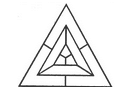Solution:

The figure may be labelled as shown.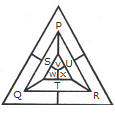The spaces P, Q and R have to be shaded by three different colours definitely (since each of these three spaces lies adjacent to the other two).

Now, in order that no two adjacent spaces be shaded by the same colour, the spaces T, U and S must be shaded with the colours of the spaces P, Q and R respectively.

Also the spaces X, V and W must be shaded with the colours of the spaces S, T and U respectively i.e. with the colours of the spaces R, P and Q respectively. Thus, minimum three colours are required.

QUESTION: 12

Find the number of triangles in the given figure.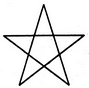Solution:

The figure may be labelled as shown.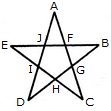The simplest triangles are AJF, FBG, GCH, HDI and IEJ i.e. 5 in number.

The triangles composed of three components each EBH, AIC, EFC, ADG and BJD i.e. 5 in number.

Thus, there are 5 + 5 = 10 triangles in the figure.

QUESTION: 13

Find the number of triangles in the given figure.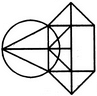Solution:

The figure may be labelled as shown.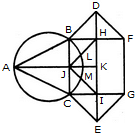The simplest triangles are ABJ, ACJ, BDH, DHF, CIE and GIE i.e. 6 in number.

The triangles composed of two components each are ABC, BDF, CEG, BHJ, JHK, JKI and CJI i.e. 7 in number.

There is only one triangle JHI which is composed of four components.

Thus, there are 6 + 7 + 1 = 14 triangles in the given figure.

QUESTION: 14

Find the minimum number of straight lines required to make the given figure.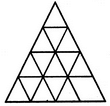Solution:

The figure may be labelled as shown.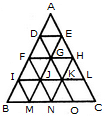The horizontal lines are DE, FH, IL and BC i.e. 4 in number.

The slanting lines are AC, DO, FN, IM, AB, EM and HN i.e. 7 in number.

Thus, there are 4 + 7 = 11 straight lines in the figure.

QUESTION: 15

Find the number of triangles in the given figure.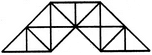Solution:

The figure may be labelled as shown.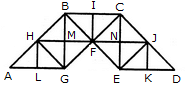The simplest triangles are AHL, LHG, GHM, HMB, GMF, BMF, BIF, CIF, FNC, CNJ, FNE, NEJ, EKJ and JKD i.e. 14 in number.

The triangles composed of two components each are AGH, BHG, HBF, BFG, HFG, BCF, CJF, CJE, JEF, CFE and JED i.e. 11 in number.

The triangles composed of four components each are ABG, CBG, BCE and CED i.e. 4 in number.

Total number of triangles in the given figure = 14 + 11 + 4 = 29.Use Code STAYHOME200 and get INR 200 additional OFF Use Coupon Code